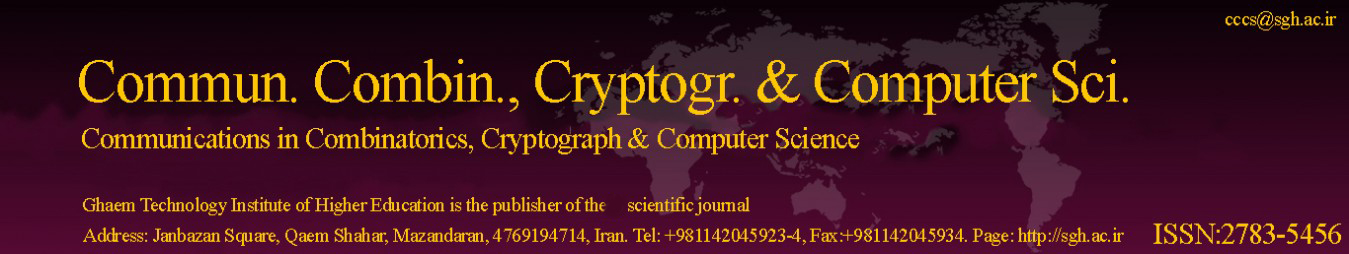Original Research Article

Article volume = 2022 and issue = 1

Pages: 1–6

Article publication Date: November 21, 2022

# Numerical solutions of Fokker-Planck Equation based on Hermite functions

#### Manoochehr Khasi(a) and Fereshteh Samadi(b)

(a) Iran University of Science and Technology, Tehran, Iran.

(b) Department of Mathematics, Payame Noor University (PNU), P.O. Box 19395-3697, Tehran, Iran

##### Abstract:

In this research study, a spectral method based on Hermite functions is considered to solve the Fokker--Planck equation which is an equation governed by the time evolution of probability density function of the stochastic processes. Spatially the equation is discretized using a Galerkin method based on Hermite functions and the system of a first--order ordinary differential equation is obtained. Using an eigenvalue decomposition technique, the equation is transformed into a set of independent ordinary differential equations that can be solved analytically. Some numerical examples are included to show the accuracy and efficiency of the proposed approach.

##### Keywords:

Fokker-Planck equation, Hermite functions, spectral Galerkin method.

##### References:
•  Johnson Fok, Benyu Guo, and Tao Tang. Combined hermite spectral-finite difference method for the fokker-planck equation. Mathematics of computation, 71(240):1497–1528, 2002. 1
•  Ben-yu Guo and Tian-jun Wang. Composite generalized laguerre-legendre spectral method with domain decomposition and its application to fokker-planck equation in an infinite channel. Mathematics of Computation, 78(265):129–151, 2009. 1
•  Jing Guo, Cheng Wang, Steven M Wise, and Xingye Yue. An h2 convergence of a second-order convex-splitting, finitedifference scheme for the three-dimensional cahn–hilliard equation. Communications in Mathematical Sciences, 14(2):489–515, 2016. 1
•  Hannes Risken. Fokker-planck equation. Springer, 1996. 1
•  Jie Shen, Tao Tang, and Li-Lian Wang. Spectral methods: algorithms, analysis and applications, volume 41. Springer Science & Business Media, 2011. 2